Angle Sum Property and Axioms - Lines and Angles, Class 9, Mathematics

# Angle Sum Property and Axioms - Lines and Angles, Class 9, Mathematics - Extra Documents & Tests for Class 9

AXIOM-1 : If a ray stands on a line, then the sum of two adjacent angles so formed is 180�.
This gives us another definition of linear pair of angles – when the sum of two adjacent angles is 180�, then they are called as linear pair of angles. In figure, a ray PQ standing on a line ∠forms a pair of adjacent angles ∠ BPQ and ∠ QPA.
The sum of the measure of the two angles is a straight angle which is equal to 180�.
The ray PB and PA are opposite rays. Thus, the two angles form a linear pair of angles. The above axiom can be stated in the reverse way as below: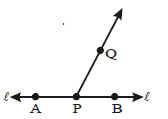AXIOM-2 : If the sum of two adjacent angles is 180�, then the non-common arms of the angles form a line. In figure, angles ∠BAC and ∠CAD are adjacent angles such that ∠BAC + ∠CAD = 180�

Then, we have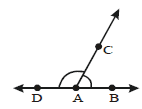Thus, ∠BAD is a straight angle. Therefore, AB and AD are in one line. The axiom 1 and 2 are called linear pair axioms. The two axioms are reverse of each other.

(x) Vertically Opposite Angles :- If two lines intersect each other then, the pairs of opposite angles formed are called vertically opposite angles. Two lines AB and CD intersect at point O.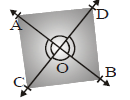Then, two pairs of vertically opposite angles are formed.
One pair is ∠AOD & ∠BOC. The other pair is ∠AOC & ∠BOD.

THEOREM-1 :- If two lines intersect each other, then the vertically opposite angles are equal.
Given : Two lines AB and CD intersect at a point O.
Two pairs of vertically opposite angles are :
(i) ∠AOC and ∠BOD
(ii) ∠AOD and ∠BOC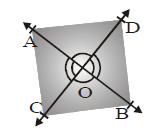To Prove : (i) ∠AOC =∠BOD
(ii) ∠AOD = ∠BOC i.e. two pairs of vertically opposite equal angles.

Proof :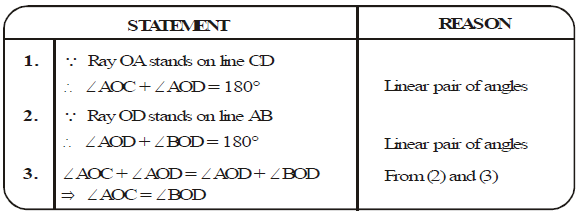Ex. In figure, ∠AOC = 50o and ∠BOC = 20o , find ∠AOB

Sol.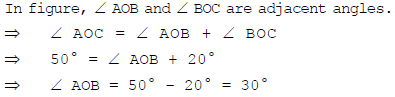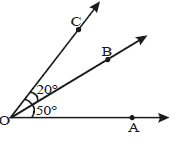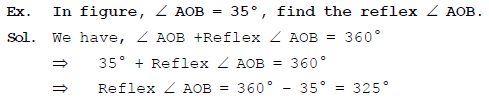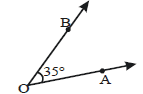Ex. In figure,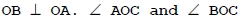are complementary angles. Find the value of x and hence find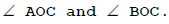Sol. In figure, We have, ∠AOC + ∠BOC = 90�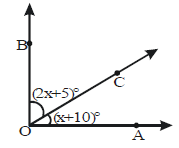⇒ (x+ 10)� + (2x + 5)� = 90�
⇒ 3x + 15� = 90�
⇒ 3x = 90� – 15� = 75�
⇒ x = 25�
Now, ∠AOC = (25 + 10)� = 35� and ∠BOC = {2 � 25 + 5}� = 55�

Ex. In figure, ray AD stands on the line CB, BAD = (2x + 10)� and CAD = (5x + 30)�, find the value of x and also write BAD and CAD.
Sol.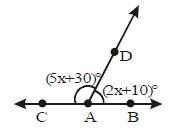⇒ (2x + 10)� + (5x + 30)� = 180�

⇒ 7x + 40� = 180�

⇒ 7x = 180� – 40�

⇒ 7x = 140�

⇒ x = 140/7

⇒ x = 20

Now, ∠BAD = (2 � 20 + 10)� = 50�

and ∠CAD = (5 � 20 + 30)� = 130�

Ex. In figure, ray OC stands on the line AB and ∠BOC = 125�. Find reflex ∠AOC.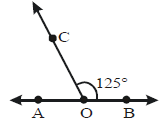Sol. In figure, ∠AOC and ∠BOC are linear pair angles.

⇒ ∠AOC + ∠BOC = 180�

⇒ ∠AOC + 125� = 180�

⇒ ∠AOC = 180� – 125� = 55� (∵ ∠BOC = 125�)

Now, Reflex ∠AOC = 360� – ∠AOC = 360� – 55� = 305�

CORRESPONDING ANGLES AXIOM:- If a transversal intersects two parallel lines, then each pair of corresponding angles are equal.
Conversely, if a transversal intersects two lines, making a pair of equal corresponding angles, then the lines are parallel.
By using the above axiom, we can now deduce the other facts about parallel lines and their transversal.

THEOREM-2 :- If a transversal intersects two parallel lines, then each pair of alternate interior angles is equal.

Given : AB and CD are two parallel lines and a transversal EF intersects them at point G and H respectively. Thus, the alternate 1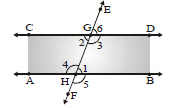interior angles are ∠2 and∠1, and ∠3 and ∠4.

To Prove : ∠1 = ∠2 and ∠3 = ∠4

Proof :

 STATEMENT REASON 1.2.3.4.5.6. ∠2 =∠6∠1 = ∠6∠2 = ∠6 and ∠1 = ∠6 ⇒1 = ∠2Similarly ∠4 = ∠5∠3 = ∠5∠4 = ∠5 and ∠3 = ∠5 ⇒3 = ∠4 Vertically opposite anglesBy corresponding angles axiomFrom equation (1) and (2)Vertically opposite anglesBy corresponding angles axiomFrom equation (4) and (5)

Hence, proved.

THEOREM-3 (converse of theorem of 2) :- If a transversal intersects two lines in such a way that a pair of alternate interior angles are equal, then the two lines are parallel.

Given : A transversal 't' intersects two lines AB and CD at P and Q respectively such that ∠1 and ∠2 are a pair of alternate interior angles and ∠1 = ∠2.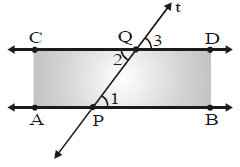To Prove : AB║CD

Proof :

 STATEMENT REASON 1 ∠2 = ∠3 Vertically opposite angles 2 ∠1 = ∠2 Given 3 ∠2 = ∠3 and ∠1 = ∠2 ⇒ ∠1 = ∠3 From (1) and (2) 4 AB║CD By converse of corresponding angles axiom

Hence, proved.

THEOREM-4 :- If a transversal intersects two parallel lines, then each pair of consecutive interior angles are supplementary.
Given : AB and CD are two parallel lines. Transversal "t" intersects AB at P and CD at Q, making two pairs of
consecutive interior angles, ∠1, ∠2 and ∠3, ∠4.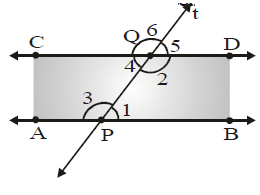To Prove :

(i) ∠1 + ∠2 = 180� and

(ii) ∠3 + ∠4 = 180�

Proof :

 STATEMENT REASON 1. ∵ AB ║ CD∴ ∠1 = ∠5 Corresponding ∠s axiom 2. ∠5 + ∠2 = 180� Linear pair of angles 3. ∠1 + ∠2 = 180� From (1) and (2) 4. ∵ AB ║ CD∴ ∠3 = ∠6 Corresponding ∠s axiom 5. ∠6 + ∠4 = 180� Linear pair of angles 6. ∠3 + ∠4 = 180� From (4) and (5)

Hence, proved.

THEOREM-5 (converse of theorem 4) :- If a transversal intersects two lines in such a way that a pair of consecutive interior angles are supplementary, then the two lines are parallel.

Given : A transversal t intersect two lines AB and CD at P and Q respectively such that ∠1 and ∠2 are a pair of
consecutive interior angles, and ∠1 + ∠2 = 180�.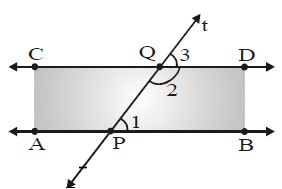To Prove : AB║CD

Proof :

 STATEMENT REASON 1. ∠1 + ∠2 = 180� Given 2. ∠2 + ∠3 = 180� Linear pair of angles 3. ∠1 + ∠2 = ∠2 + ∠3⇒ ∠1 = ∠3 From (1) and (2) 4. ⇒ AB ║ CD By converse of corresponding angles axiom

Hence, proved.

THEOREM-6 :- If two lines are parallel to the same line, they will be parallel to each other.

Given : Line m║ line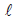and line n ║ line.

To Prove : Line m║ line n.

Construction : Draw a line t transversal for the lines, m and n.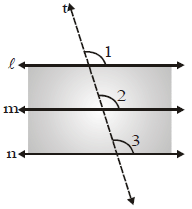Proof :

 STATEMENT REASON 1. ∵  m║∴ ∠1 = ∠2 Corresponding angles 2. ∵ n║∴ ∠1 = ∠3 Corresponding angles 3. ∠1 =∠2 and ∠1=∠3 ⇒ ∠2=∠3 From (1) and (2) 4. ⇒ m║n By converse of corresponding angles axiom

Hence, proved.

ANGLE SUM PROPERTY OF A TRIANGLE

In previous classes, we have learnt that the sum of the three angles of a triangle is 180�. In this section, we shall prove this fact as a theorem.

THEOREM-7 :- The sum of all the angles of a triangle is 180�.
Given : In a Δ PQR, P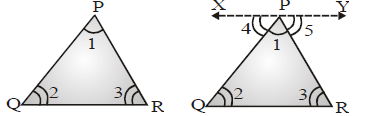∠1, ∠2 and ∠3 are the angles of Δ PQR.
To Prove : ∠1 + ∠2 + ∠3 = 180�
Construction :
Draw a line XPY parallel to QR through the opposite vertex P.

Proof :

 STATEMENT REASON 1. ∠4 + ∠1 + ∠5 = 180� XPY is a line 2. ∵ XPY ⊥ QR∴ ∠4 = ∠2 and∠5 = ∠3 Alternate interior angles 3. ∠2 + ∠1 +∠3 = 180� or ∠1 + ∠2 + ∠3 = 180� From (1) and (2)

Hence, proved.

EXTERIOR ANGLE OF A TRIANGLE
Consider the ΔPQR. If the side QR is produced to point S, then ∠PRS is called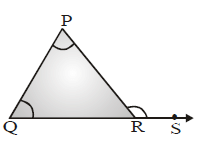an exterior angle of ΔPQR.
The ∠PQR and ∠QPR are called the two interior opposite angles of the exterior ∠PRS.

THEOREM-8 :- If a side of a triangle is produced, then the exterior P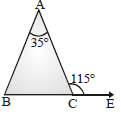angle so formed is equal to the sum of the two interior opposite angles.
Given : In a Δ PQR, ∠1, ∠2 and ∠3 are the angles of ΔPQR, side QR is produced to S and exterior angle ∠PRS = ∠4.
To Prove : ∠4 = ∠1 + ∠2
Proof :

 STATEMENT REASON 1. ∠3 + ∠4 = 180� Linear pair of angles 2. ∠1 + ∠2 + ∠3 = 180� The sum of all the angles of a triangles is 180� 3. ∠3 + ∠4 = ∠1 + ∠2 + ∠3⇒∠4 = ∠1 + ∠2. From (1) and (2)

Hence, proved.

The document Angle Sum Property and Axioms - Lines and Angles, Class 9, Mathematics | Extra Documents & Tests for Class 9 is a part of the Class 9 Course Extra Documents & Tests for Class 9.
All you need of Class 9 at this link: Class 9

## Extra Documents & Tests for Class 9

1 videos|228 docs|21 tests

## FAQs on Angle Sum Property and Axioms - Lines and Angles, Class 9, Mathematics - Extra Documents & Tests for Class 9

 1. What is the Angle Sum Property?Ans. The Angle Sum Property states that the sum of all the angles in a triangle is always equal to 180 degrees. This property holds true for all types of triangles, including equilateral, isosceles, and scalene triangles.
 2. How can I prove the Angle Sum Property?Ans. The Angle Sum Property can be proved using the concept of parallel lines and transversals. By drawing a line parallel to one side of the triangle and intersecting the other two sides, we can create alternate interior angles. By using the fact that the sum of alternate interior angles is 180 degrees, we can establish that the sum of angles in a triangle is 180 degrees.
 3. What are axioms in the context of Lines and Angles?Ans. Axioms are statements or principles that are considered to be self-evidently true and are used as a starting point for deriving other mathematical statements. In the context of Lines and Angles, axioms are fundamental assumptions or properties that help establish the basic principles of geometry, such as the existence of a unique line passing through two distinct points.
 4. Can the Angle Sum Property be extended to other polygons?Ans. No, the Angle Sum Property only holds true for triangles. The sum of angles in any polygon can be found using the formula (n-2) * 180, where n represents the number of sides of the polygon. For example, the sum of angles in a quadrilateral (4-sided polygon) is (4-2) * 180 = 360 degrees.
 5. How is the Angle Sum Property useful in solving geometry problems?Ans. The Angle Sum Property is a fundamental concept in geometry and is useful in many problem-solving scenarios. It can be used to find missing angles in triangles, determine whether a given set of angles can form a triangle, prove various geometric theorems, and establish relationships between angles in different shapes. Understanding the Angle Sum Property enables students to solve complex geometry problems efficiently.

## Extra Documents & Tests for Class 9

1 videos|228 docs|21 testsExplore Courses for Class 9 examSignup to see your scores go up within 7 days! Learn & Practice with 1000+ FREE Notes, Videos & Tests.
10M+ students study on EduRev
Track your progress, build streaks, highlight & save important lessons and more!
Related Searches

,

,

,

,

,

,

,

,

,

,

,

,

,

,

,

,

,

,

,

,

,

,

,

,

,

,

,

;